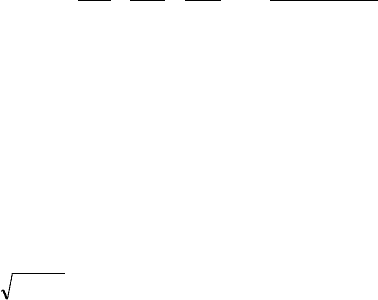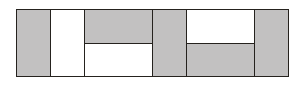# MATH 111 Study Guide - Quiz Guide: Mathematical Induction, Inductive Reasoning, The Sequence

190 views16 pages111 Test 5 preparation problems 2015-16
1
Math 111 Test 5 Preparation Problems Feb. 2016
Test 5 (Thursday Feb 25) will have 3 questions. The first two are induction questions. To get full marks
on these your exposition will have to be of good quality (though that does not mean it has to be long).
The third question will ask you to formulate a recursive equation for a sequence which I will define. This
is the other side of the inductive process in which you are asked to use inductive reasoning to formulate
the hypothesis you will then want to establish. In a sense this embraces all the work we have done (and
will continue to do) since the beginning of term. You have seen a number of examples of these and
following the induction examples below, I give you a bit of a list. Finally this document ends with
solutions to Test 5 from the last two years but note that in 2013-14 Test 5 was a week later and a couple
of its questions have been removed as they belonged to this year’s Test 6.
1. Find a simple expression for the following sum.
 
1212
1
75
1
53
1
31
1
nn
...
.
[Work out the cases n = 1, n = 2, n = 3, and see the pattern.] Then use mathematical induction to prove
that the formula holds for all n.
2. Consider the recursive equation: tn+1 = 4tn 3tn1 t0 = 0, t1 = 1.
Generate a few terms of the sequence and observe that each term is one more than three times the
preceding term. Use mathematical induction to prove that this continues to hold.
3. Consider the recursive equation xn+1 =
n
x2
x0 = 1.
Show that the sequence is increasing.
4. What is the sum of the first n Fibonacci numbers? Make a conjecture
and use mathematical induction to establish it.
5. Find a formula for the nth power of the matrix A =
100
110
211
and use mathematical induction to
show that it holds. [Too long and hard for a test but a good prep problem.]
Unlock document

This preview shows pages 1-3 of the document.
Unlock all 16 pages and 3 million more documents.

Already have an account? Log in111 Test 5 preparation problems 2015-16
2
Now some examples on formulating linear recursive equations (no solutions given).
1.[Ch4 probs #1] I want to construct a train using cars which are either length 1 or of length 3. Let xn be
the number of different trains of length n. For example, x6 = 6. Find a recursive formula that would
allow you to generate the sequence xn from a few starting values.
2. [Ch4 probs #3] I want to construct a train of total length n using cars which are either of length 1 or 2.
Suppose there are two different kinds of cars of length 1 and three different kinds of cars of length 2. Let
n
t
be the number of trains of length n. Find a linear recursion for
n
t
.
3. [Assign 6 #5] I want to pave a 2×n rectangle with 1×2 blocks
which come in two colours, white and grey. Let wn be the
number of different ways this can be done. For example, it can
be shown that w8 = 8704 and the diagram at the right displays
one of these pavings. Find a recursive equation for wn and
provide enough initial conditions so that the values of the wn will
be determined by the recursion.
4. Suppose I draw n lines in the plane, no two parallel, no three concurrent. Let Rn be the number of
regions created. Find a recursive formula for Rn. [Answer: Rn+1 = Rn + n + 1 because if I have n lines, a
new line will cross all n existing lines and that will cut n + 1 old regions in two. See Ex 458: Regions in
a circle.”]
5. Hitting 10 (Ex 441). You toss a coin repeatedly. If heads is worth 1 and tails is worth 2, and you add
up the score as you go along, what is the probability that you’ll hit 10? Formulate a second order
recursive equation which would allow you to calculate this probability.
6. Two heads in a row (Ex457). A fair coin is tossed until two consecutive heads appear. This might take
2 tosses, or three, or four, etc. Let pn be the probability that it will take exactly n tosses. Thus p3 = 1/8
because only 1 of the 8 possible sequences of H and T gets two consecutive heads for the first time
exactly on the 3rd toss (that’s THH). Find a recursive formula for the sequence pn.
Unlock document

This preview shows pages 1-3 of the document.
Unlock all 16 pages and 3 million more documents.

Already have an account? Log in111 Test 5 preparation problems 2015-16
3
Solutions.
1. Find a simple expression for the following sum.
.
[Work out the cases n = 1, n = 2, n = 3, and see the pattern.] Then use mathematical induction to prove
that the formula holds for all n.
By working with the first few cases we guess the formula
P(n):
  
121212
1
...
75
1
53
1
31
1
n
n
nn
and we have called this formula P(n).
1) Establish P(1): This is the equation
12
1
31
1
and this clearly holds.
2) Now assume that P(n) holds. To establish P(n+1), we have to show
 
1)1(2
1
1)1(21)1(2
1
1212
1
...
75
1
53
1
31
1
n
n
nnnn
There are n+1 terms on the left. The induction hypothesis tells us that the sum of the first n terms is
n/(2n+1). Thus the left hand side can be written
 
3212
1
12
nnn
n
 
 
3212
132
nn
nn
 
3212
132 2
nn
nn
 
3212
)1)(12(
nn
nn
32
1
n
n
And this is the same as the right hand side of P(n+1). We can conclude that the formula holds for all n.
Unlock document

This preview shows pages 1-3 of the document.
Unlock all 16 pages and 3 million more documents.

Already have an account? Log in

# Get access

Grade+
\$10 USD/m
Billed \$120 USD annually
Homework Help
Class Notes
Textbook Notes
40 Verified Answers
Study Guides
1 Booster Class